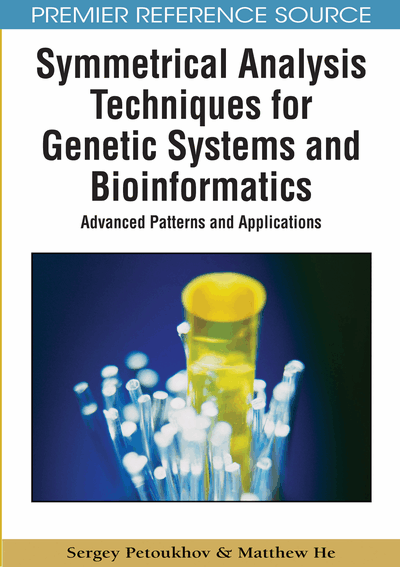# Multidimensional Numbers and the Genomatrices of Hydrogen Bonds

Sergey Petoukhov (Russian Academy of Sciences, Russia) and Matthew He (Nova Southeastern University, USA)
DOI: 10.4018/978-1-60566-124-7.ch009
Available
\$37.50
No Current Special Offers

## Abstract

This chapter returns to the kind of numeric genetic matrices, which were considered in Chapter 4-6. This kind of genomatrices is not connected with the degeneracy of the genetic code directly, but it is related to some other structural features of the genetic code systems. The connection of the Kronecker families of such genomatrices with special categories of hypercomplex numbers and with their algebras is demonstrated. Hypercomplex numbers of these two categories are named “matrions of a hyperbolic type” and “matrions of a circular type.” These hypercomplex numbers are a generalization of complex numbers and double numbers. Mathematical properties of these additional categories of algebras are presented. A possible meaning and possible applications of these hypercomplex numbers are discussed. The investigation of these hyperbolic numbers in their connection with the parameters of molecular systems of the genetic code can be considered as a continuation of the Pythagorean approach to understanding natural systems.
Chapter Preview
Top

## Introduction And Background

The discovery of quaternions by Hamilton in 1843 has led to many important consequences for all mathematical natural sciences (Kline, 1980). Hamilton believed that this discovery was the most significant achievement of his life though his name is connected in modern science with many other essential notions and formalisms: the famous equation by Hamilton, which is called “the canonical equation of mechanics” and which underlies the whole of theoretical physics; “functions by Hamilton”; “Hamiltonians”, etc. After the discovery of quaternions, Hamilton devoted to their study last 20 years of his life, specially having refused a post as the president of academy of sciences of Ireland. Within these years he published 109 scientific works devoted to quaternions, including two fundamental monographs. Only in the XIX-th century almost 600 scientific works were published and which were devoted to the theory of these hypercomplex numbers and to their successful applications to various problems in physics, geometry, the theory of numbers, etc. One of the main consequences was the understanding that various natural systems can possess their own algebra or that they can be connected closely with various systems of multi-dimensional numbers.

It seems an important task to investigate from different viewpoints is what systems of multi-dimensional numbers (or what types of multi-dimensional algebras) are connected or can be connected with ensembles of parameters of the genetic code and with relevant bioinformation spaces. And what symmetries and patterns are typical for these numerical systems and for their matrix forms of presentation. This chapter describes some results of such investigations.

A development of the notion of “number” has a long history. This history is described in many monographs and it abounds with interesting discoveries and generalizations, many of which are known widely. An appearance of two-dimensional numbers, which were named “complex numbers” by “the king of mathematics” C. Gauss at the end of the XVIII century, was one of them. This kind of numbers utilized the notion of “imaginary unit”, which was proposed in the middle of XVI century by the Italian doctor, the mathematician and the designer of mechanisms (in particular, a cardan shaft) G. Cardano. Similarly to real numbers, complex numbers possess the commutative property on multiplication: the result of multiplication of two complex numbers does not depend on the order of factors. This important property allowed developing the theory of functions of complex variables, which plays a significant role in modern science. These two-dimensional numbers have appeared very useful not only in the sphere of pure mathematics, but in many applied fields as well. These complex numbers are the mathematical basis of quantum mechanics and of many other branches of mathematical natural sciences.

There is no doubt that development of formalized theories depends highly on those mathematical notions and instruments, on which they are based. Two-dimensional complex numbers, which are a sum of real item and imaginary item, have appeared as magic instruments for the development of theories and calculations in the field of problems of heat, light, sounds, vibrations, elasticity, gravitation, magnetism, electricity, liquid streams, and phenomena of a micro-world. Therefore attempts were repeatedly undertaken to construct the generalized numbers with greater dimension by means of inclusion of additional items into the structure of complex numbers. Those “numbers”, which can be constructed by means of the addition of imaginary units ik = -1 to real unit, were named “hypercomplex numbers”. As a result of such attempts, W.Hamilton has created 4-dimensional numbers, which contain one real unit and three imaginary units and which were named quaternions. Quaternions by Hamilton do not possess the commutative property.

Hypercomplex numbers in their modern comprehension can contain not only real and imaginary units but also a special unit ek, which possesses the property ek2 = +1 but which differs from real unit. In this chapter we shall name these units ek as “semi-imaginary units” because in the multiplication tables of matrions (Figures 5 and 10) they possess some properties of real unit and of imaginary unit simultaneously. A review of many known kinds of multi-dimensional numbers is presented on the websites http://hypercomplex.xpsweb.com. An important place among hypercomplex numbers belong to those numbers which possess the commutative property and the associative property simultaneously. Matrions, which are described in this chapter, belong to this category of hypercomplex numbers.

## Complete Chapter List

Search this Book:
Reset